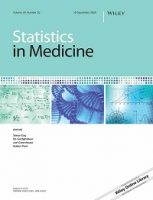# Lay abstract for Statistics in Medicine article: Comments on “Sample size formula for a win ratio endpoint” by R. X. Yu and J. GanjuEach week, we publish lay abstracts of new articles from our prestigious portfolio of journals in statistics. The aim is to highlight the latest research to a broader audience in an accessible format.

This Letter to the Editor featured today is from Statistics in Medicine and is now available to read in full here.

Gasparyan, S.B., Kowalewski, E.K. and Koch, G.G. (2022), Comments on “Sample size formula for a win ratio endpoint” by R. X. Yu and J. Ganju. Statistics in Medicine, 41: 2688-2690. https://doi.org/10.1002/sim.9379

The win ratio endpoints are ordinal endpoints (with outcomes that can be compared but for which arithmetic operations are not applicable) for two group trials. They are produced by comparing every patient in the intervention group with every patient in the control group. If a comparison results in a better outcome for the patient in the intervention group, there is a “win” for the patient in the intervention group; and if such a comparison results in a worse outcome, then there is a “loss”. From comparisons of all patients in the intervention group with all patients in the control group, the win ratio statistic is constructed as the total number of wins divided by the total number of losses for the intervention group. This statistic disregards ties and can potentially exaggerate the degree of dissimilarity of two treatment groups that are being compared when ties are substantial. Alternatively, the total number of wins for the intervention group plus half of the total number of ties, divided by the total number of losses plus half of the total number of ties, produces the win odds statistic. By including ties in its definition, the win odds statistic can be a more realistic way of comparing two distributions.

An important question is how to design studies based on win ratio or win odds tests. That is, given the value of the win odds/win ratio and the required power, what is the sample size needed for a two group trial to detect the given value? Two recent publications, one in Statistics in Medicine and the other in the Journal of Biopharmaceutical Statistics, provide sample size calculation formulas for the tests based on the win ratio and the win odds, respectively. In this letter we compare these two formulas. Both are general formulas that can incorporate unbalanced randomization and ties. We note that if there are no ties, the win ratio and the win odds are the same, and both formulas give similar results in terms of required sample sizes. While in the presence of ties, the main difference is how the alternative hypothesis is formulated, in terms of a win odds or a win ratio. For a categorical ordinal outcome, if the expected number of ties or the expected proportion of each outcome (for both groups combined) are known in advance, then the required sample size can be decreased for both the win ratio and win odds tests.